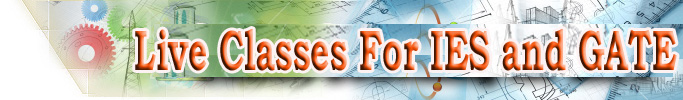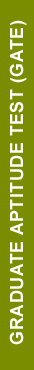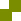﻿ Engineering service exam Syllabus for ECE | ECE IES Reference books | IES and ESE reference books for Electronics and Communication Engineering
 Join  our Virtual Interactive Classroom NOW! Try out our Sample Test Watch our Sample Video Attend our  Orientation Programme Call: +91 9000018804Home | Institute | GATE | IES | Virtual Classroom | Courses | Faculty | Resources | Feedbacks | Contact UsGATE - QUESTION PATTERN

The GATE examination consists of a single paper of 3 hours duration which contains 65 questions carrying a maximum of 100 marks, out of which 10 questions carrying total of 15 marks are in General Aptitude (GA). The remaining of 85% of the total marks is devoted to the syllabus of the paper.

The question paper will consist of only objective questions. Depending on the GATE PAPER chosen by the candidate, two types of examination are conducted.

• ONLINE EXAMINATION: The examination for the papers with codes AE, AG, AR, BT, CE, CH, CY, GG, PH, MA, MN, MT, TF, XE, and XL will be carried out as Online Computer-based Test where the candidates will be shown the questions in a random sequence on a computer screen. The candidates are required to enter the answer for each question using a mouse (keyboards will be disabled).
• OFFLINE EXAMINATION: A paper based examination where the candidate will mark the correct answer out of four options in an Optical Response Sheet (ORS) by darkening the appropriate bubble with a black ink ball point pen. For CS, EC, EE, IN, ME, and PI, the candidates will be given the questions printed on a paper, and they have to mark the correct choice on an Optical Response Sheet (ORS) by darkening the appropriate bubble against each question using a black ink ball point pen.

TYPES OF QUESTIONS:

The GATE Examination would contain questions of four different types in various papers:

1. Multiple choice questions carrying 1 or 2 marks each. Each of  the multiple choice objective questions in all papers and sections will contain four answers,  of which one correct answer is to be marked.
2. Common data questions (which are also multiple choice questions), where TWO successive questions use the same set of input data. Two questions are linked to a common data problem, passage and the like. Each question is independent and its solution is obtainable from the above problem data or passage directly. (Answer of the previous question is not required to solve the next question). Each question under this group will carry two marks.
3. Linked answer questions (which are also multiple choice questions), where the answer to the first question in the pair is required to answer its successor. These questions are of problem solving type. A problem statement is followed by two questions based on the problem statement. The two questions are designed such that the solution to the second question depends upon the answer to the first one. In other words, the first answer is an intermediate step in working out the second answer. Each question in such ‘linked answer questions’ will carry two marks.
4. Numerical answer questions, where the answer is a number, to be entered by the candidate using the mouse and a virtual keypad that will be provided on the screen.

DESIGN OF QUESTIONS:

The questions in a paper may be designed to test the following abilities:

1. Recall: These are based on facts, principles, formulae or laws of the discipline of the paper. The candidate is expected to be able to obtain the answer either from his/her memory of the subject or at most from a one-line computation.
2. Comprehension: These questions will test the candidate’s understanding of the basics of his/her field, by requiring him/her to draw simple conclusions from fundamental ideas.
3. Application: In these questions, the candidate is expected to apply his/her knowledge either through computation or by logical reasoning.
4. Analysis and Synthesis:  These can be linked answer questions, where the answer to the first question of the pair is required in order to answer its successor. Or these can be common data questions, in which two questions share the same data but can be solved independently of each other.

The questions based on the above four logics may be a mix of single stand alone statement/phrase /data type questions, combination of option codes type questions or match items type questions.